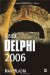Boolean and Bitwise Operators

Delphi has four Boolean operators: not, and, or, xor. Boolean operators are used to create more complex conditions. All Boolean operators work with Boolean values and return a Boolean value as the result. Bitwise operators work with integer values and return an integer value as the result. Delphi has six bitwise operators: not, and, or, xor, shl, shr.

The not Operator

The Boolean not operator is a unary operator used to invert a Boolean value. The following table shows the results of the not operator.

Table 3-2: Boolean not operator

Expression

not Expression

True

False

False

True

Listing 3-6: Using the Boolean not operatorprogram Project1; {\$APPTYPE CONSOLE} uses   SysUtils; var   Test: Boolean; begin   WriteLn('Default Boolean Value: ', Test);   WriteLn('Inverted Boolean Value: ', not Test);   ReadLn; end.The first WriteLn statement always displays False because Delphi automatically initializes global Boolean variables to False.

The Boolean not operator is often used in an if-then statement as a more elegant way of testing whether the expression evaluates to False:

var   B: Boolean; begin   if B = False then     WriteLn('Not OK');   if not B then     WriteLn('Not OK');   ReadLn; end.

The same elegance can be used when testing whether the expression evaluates to True:

var   B: Boolean; begin   B := True;   if B = True then     Writeln('OK');   if B then     WriteLn('OK');   ReadLn; end.

The bitwise not operator is used to perform a bitwise negation of an integer value. The following table shows the results of the bitwise not operator.

Table 3-3: Bitwise not operator

Bit

not Bit

1

0

0

1

The and Operator

The Boolean and operator is a binary operator that returns True only if both tested expressions evaluate to True. The following table shows the result of using the and operator.

Table 3-4: Boolean and operator

Expression1

and Expression2

Result

True

True

True

True

False

False

False

True

False

False

False

False

Let's say that we need to write a piece of code that can only work properly if the user enters a number in the –10 to 10 range. To make sure the entered value is in this range, we can use either two nested if-then statements or one if-then statement that uses the Boolean and operator.

Listing 3-7: Using the Boolean and operatorprogram Project1; {\$APPTYPE CONSOLE} uses   SysUtils; var   Num: Integer; begin   Write('Enter a number from -10 to 10: ');   ReadLn(Num);   if (Num >= -10) and (Num <= 10) then     WriteLn('Range Test OK.')   else     WriteLn('You''ve entered an invalid value!');   ReadLn; end.Note Both expressions must be separated from the reserved word and by parentheses. This is necessary because the Boolean and operator has higher precedence than the relational operators. If you forget the parentheses, you will get an "Incompatible types" compiler error. Delphi generates this error because, without the parentheses, the compiler interprets the and operator as the bitwise and operator. Things go awry because the bitwise and operator returns an integer and the if-then statement expects a Boolean value.

The following table shows the results of the bitwise and operator.

Table 3-5: Bitwise and operator

Bit1

and Bit2

Result

0

0

0

0

1

0

1

0

0

1

1

1

The or Operator

The Boolean or operator is used to test whether at least one expression evaluates to True. The only time the or operator returns False is when both expressions evaluate to False. The following table shows the results of the or operator.

Table 3-6: Boolean or operator

Expression1

or Expression2

Result

True

True

True

False

True

True

True

False

True

False

False

False

Listing 3-8: Using the Boolean or operatorprogram Project1; {\$APPTYPE CONSOLE} uses   SysUtils; var   c: Char; begin   Write('Format C: (Yes/No): ');   ReadLn(c);   { See if the user selected yes }   if (c = 'y') or (c = 'Y') then     WriteLn('Formatting... ;)')   else if (c = 'n') or (c = 'N') then     WriteLn('Too bad...')   else     WriteLn('You have to type "y" for yes and "n" for no.');   ReadLn; end.The following table shows the results of the bitwise or operator.

Table 3-7: Bitwise or operator

Bit1

or Bit2

Result

0

0

0

1

0

1

0

1

1

1

1

1

The xor Operator

The xor (exclusive or) operator is used to test whether only one expression evaluates to True. If both expressions evaluate to either True or False, the xor operator returns False. The Boolean xor operator isn't used often, but the bitwise xor operator is used by some programmers to optimize code. The following table shows the results of both Boolean and bitwise xor operators.

Table 3-8: Boolean and bitwise xor operators

Expression1 (Bit1)

xor Expression 2 (Bit2)

Result

True (1)

True (1)

False (0)

True (1)

False (0)

True (1)

False (0)

True (1)

True (1)

False (0)

False (0)

False (0)

The shr and shl Operators

The shr (shift right) and shl (shift left) operators shift bits in integer values. The shr and shl operators are often used as a substitute for division or multiplication by 2n since both operations tend to execute slightly faster than ordinary multiplication and division.

To fully understand how the shl and shr operators function, we have to take a brief look at the binary representation of numbers. For example, let's multiply and divide the number 4 by 2. The binary representation of 4 is 0100. To multiply 4 by 2, we shift its bits one place to the left using the shl operator (4 shl 1). The result is 1000, or decimal 8. To divide 4 by 2, we shift its bits one place to the right using the shr operator (4 shr 1). The result is 0010, or decimal 2.

Listing 3-9: Using the shr and shl operatorsprogram Project1; {\$APPTYPE CONSOLE} uses   SysUtils; begin   WriteLn('4 / 2 = ', 4 shr 1);   WriteLn('4 * 2 = ', 4 shl 1);   WriteLn('8 / 4 = ', 8 shr 2);   WriteLn('8 * 8 = ', 8 shl 3);   ReadLn; end.Inside Delphi 2006 (Wordware Delphi Developers Library)
ISBN: 1598220039
EAN: 2147483647
Year: 2004
Pages: 212